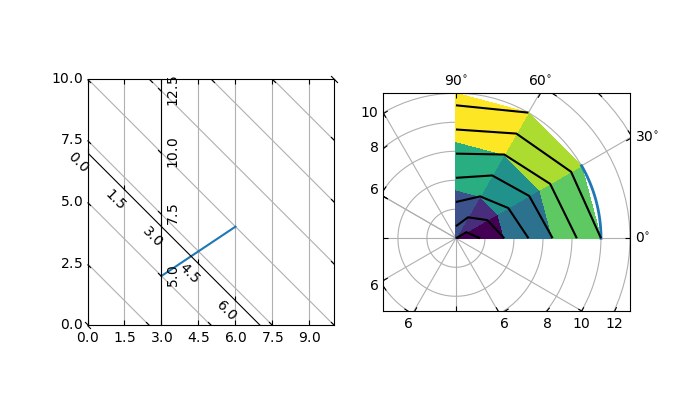# Curvilinear grid demo#

Custom grid and ticklines.

This example demonstrates how to use GridHelperCurveLinear to define custom grids and ticklines by applying a transformation on the grid. This can be used, as shown on the second plot, to create polar projections in a rectangular box.import numpy as np

import matplotlib.pyplot as plt
from matplotlib.projections import PolarAxes
from matplotlib.transforms import Affine2D

from mpl_toolkits.axisartist import angle_helper, Axes, HostAxes
from mpl_toolkits.axisartist.grid_helper_curvelinear import (
GridHelperCurveLinear)

def curvelinear_test1(fig):
"""
Grid for custom transform.
"""

def tr(x, y): return x, y - x
def inv_tr(x, y): return x, y + x

grid_helper = GridHelperCurveLinear((tr, inv_tr))

ax1 = fig.add_subplot(1, 2, 1, axes_class=Axes, grid_helper=grid_helper)
# ax1 will have ticks and gridlines defined by the given transform (+
# transData of the Axes).  Note that the transform of the Axes itself
# (i.e., transData) is not affected by the given transform.
xx, yy = tr(np.array([3, 6]), np.array([5, 10]))
ax1.plot(xx, yy)

ax1.set_aspect(1)
ax1.set_xlim(0, 10)
ax1.set_ylim(0, 10)

ax1.axis["t"] = ax1.new_floating_axis(0, 3)
ax1.axis["t2"] = ax1.new_floating_axis(1, 7)
ax1.grid(True, zorder=0)

def curvelinear_test2(fig):
"""
Polar projection, but in a rectangular box.
"""

# PolarAxes.PolarTransform takes radian. However, we want our coordinate
# system in degree
tr = Affine2D().scale(np.pi/180, 1) + PolarAxes.PolarTransform()
# Polar projection, which involves cycle, and also has limits in
# its coordinates, needs a special method to find the extremes
# (min, max of the coordinate within the view).
extreme_finder = angle_helper.ExtremeFinderCycle(
nx=20, ny=20,  # Number of sampling points in each direction.
lon_cycle=360, lat_cycle=None,
lon_minmax=None, lat_minmax=(0, np.inf),
)
# Find grid values appropriate for the coordinate (degree, minute, second).
grid_locator1 = angle_helper.LocatorDMS(12)
# Use an appropriate formatter.  Note that the acceptable Locator and
# Formatter classes are a bit different than that of Matplotlib, which
# cannot directly be used here (this may be possible in the future).
tick_formatter1 = angle_helper.FormatterDMS()

grid_helper = GridHelperCurveLinear(
tr, extreme_finder=extreme_finder,
grid_locator1=grid_locator1, tick_formatter1=tick_formatter1)
1, 2, 2, axes_class=HostAxes, grid_helper=grid_helper)

# make ticklabels of right and top axis visible.
ax1.axis["right"].major_ticklabels.set_visible(True)
ax1.axis["top"].major_ticklabels.set_visible(True)
# let right axis shows ticklabels for 1st coordinate (angle)
ax1.axis["right"].get_helper().nth_coord_ticks = 0
# let bottom axis shows ticklabels for 2nd coordinate (radius)
ax1.axis["bottom"].get_helper().nth_coord_ticks = 1

ax1.set_aspect(1)
ax1.set_xlim(-5, 12)
ax1.set_ylim(-5, 10)

ax1.grid(True, zorder=0)

# A parasite axes with given transform
ax2 = ax1.get_aux_axes(tr)
# note that ax2.transData == tr + ax1.transData
# Anything you draw in ax2 will match the ticks and grids of ax1.
ax2.plot(np.linspace(0, 30, 51), np.linspace(10, 10, 51), linewidth=2)

ax2.pcolor(np.linspace(0, 90, 4), np.linspace(0, 10, 4),
np.arange(9).reshape((3, 3)))
ax2.contour(np.linspace(0, 90, 4), np.linspace(0, 10, 4),
np.arange(16).reshape((4, 4)), colors="k")

if __name__ == "__main__":
fig = plt.figure(figsize=(7, 4))

curvelinear_test1(fig)
curvelinear_test2(fig)

plt.show()


Gallery generated by Sphinx-Gallery